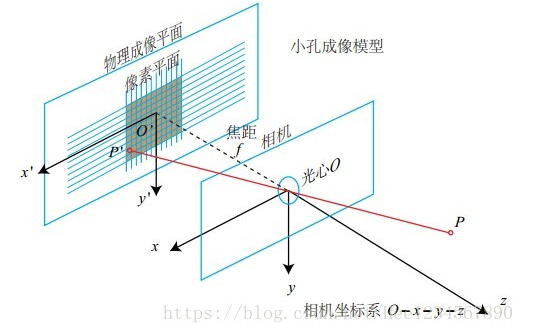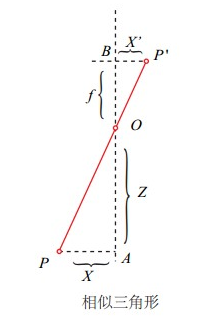## 小孔成像模型### 图像坐标系（二维坐标系）$$\frac{Z}{f}=\frac{X}{x}=\frac{Y}{y}$$

$$\left{\begin{array}{l}x = f\frac{X}{Z}\y = f\frac{Y}{Z}\z = f\end{array}\right.$$

## 齐次坐标

$$\left{ \begin{array}{l} x = f\frac{X}{Z} \ y = f\frac{Y}{Z} \ z = f \end{array} \right.$$

$$\left[\begin{array}{c}x\y\end{array}\right] \Leftrightarrow \left[\begin{array}{c}\hat{x}\\hat{y}\\hat{z}\end{array}\right] = \left[\begin{array}{ccc}f&0&0&0\0&f&0&0\0&0&1&0\end{array}\right] \left[\begin{array}{c}X\Y\Z\1\end{array}\right]$$

$$\left{\begin{array}{l}x=\frac{\hat{x}}{\hat{z}}\y=\frac{\hat{y}}{\hat{z}} \z\ne0\end{array}\right.$$

$$s(a,b,c)^T=(sa,sb,sc)^T$$

## 内参数

$$\begin{array}{c} u = \alpha\cdot x+ c_x\ v = \beta \cdot y + c_y \end{array}$$

$$\left{\begin{array}{c} u = \alpha\cdot f\frac{X}{Z}+ c_x \ v = \beta \cdot f\frac{Y}{Z} + c_y \end{array}\right. \Rightarrow \left{\begin{array}{c} u = f_x\frac{X}{Z}+ c_x \ v = f_y\frac{Y}{Z} + c_y \end{array}\right.$$

$$\left[\begin{array}{c}\mu\\nu\1\end{array}\right] = \frac{1}{Z}\left[ \begin{array}{ccc}f_x&0&c_x\0&f_y&c_y\0&0&1\end{array}\right]\left[\begin{array}{c}X\Y\Z\end{array}\right]$$

$$\left[\begin{array}{c}\mu\\nu\1\end{array}\right]=Z\left[\begin{array}{c}\mu\\nu\1\end{array}\right] =\left[ \begin{array}{ccc}f_x&0&c_x\0&f_y&c_y\0&0&1\end{array}\right]\left[\begin{array}{c}X\Y\Z\end{array}\right]$$

$$K=\left[\begin{array}{ccc}f_x&0&c_x\0&f_y&c_y\0&0&1\end{array}\right]$$
K有4个未知数和相机的构造相关，$f_x$,$f_y$和相机的焦距，像素的大小有关；$c_x$,$c_y$是平移的距离，和相机成像平面的大小有关。

## 外参数

$$p = KP$$

$$P_c = RP_w + t$$

$$\left[\begin{array}{c}X_c\Y_c\Z_c\end{array}\right] = \left[\begin{array}{ccc}R_{11}&R_{12}&R_{13}\R_{21}&R_{22}&R_{23}\R_{31}&R_{32}&R_{33}\end{array}\right]\left[\begin{array}{c}X_w\Y_w\Z_w\end{array}\right] + \left[\begin{array}{c}t_1\t_2\t_3\end{array}\right]$$

$$\left[\begin{array}{c}X_c\Y_c\Z_c\1\end{array}\right] = \left[\begin{array}{ccc}R_{11}&R_{12}&R_{13}&t_1\R_{21}&R_{22}&R_{23}&t_2\R_{31}&R_{32}&R_{33}&t_3\0&0&0&1\end{array}\right]\left[\begin{array}{c}X_w\Y_w\Z_w\1\end{array}\right]$$

$$\left[\begin{array}{c}X_c\Y_c\Z_c\1\end{array}\right] = \left[\begin{array}{cc}R&t\0^T&1\end{array}\right]\left[\begin{array}{c}X_w\Y_w\Z_w\1\end{array}\right]$$

$$T = \left[\begin{array}{cc}R&t\0^T&1\end{array}\right]$$

## 内外参数组合到一起

$$p=(\mu,\nu)$$是图像中的像点，其坐标系是像素坐标系；$$P_c=(X_c,Y_c,Z_c)$$是场景中的三维店，其坐标系是相机坐标系
$$\left[\begin{array}{c}\mu\\nu\1\end{array}\right] = \left[ \begin{array}{ccc}f_x&0&c_x\0&f_y&c_y\0&0&1\end{array}\right]\left[\begin{array}{c}X_c\Y_c\Z_c\end{array}\right]$$

$$\left[\begin{array}{c}\mu\\nu\1\end{array}\right] = \left[ \begin{array}{ccc}f_x&0&c_x&0\0&f_y&c_y&0\0&0&1&0\end{array}\right]\left[\begin{array}{c}X_c\Y_c\Z_c\1\end{array}\right]$$

## 总结

$$\left[\begin{array}{c}x\y\1\end{array}\right] = \left[\begin{array}{ccc}f&0&0&0\0&f&0&0\0&0&1&0\end{array}\right] \left[\begin{array}{c}X_c\Y_c\Z_c\1\end{array}\right]$$

$$\left[\begin{array}{c}\mu\\nu\1\end{array}\right] = \left[\begin{array}{ccc}\frac{1}{dx}&0&c_x\0&\frac{1}{dy}&c_y\0&0&1\end{array}\right] \left[\begin{array}{ccc}f&0&0&0\0&f&0&0\0&0&1&0\end{array}\right] \left[\begin{array}{c}X_c\Y_c\Z_c\1\end{array}\right]$$

$$\left[\begin{array}{c}\mu\\nu\1\end{array}\right] = \left[\begin{array}{ccc}\frac{1}{dx}&0&c_x\0&\frac{1}{dy}&c_y\0&0&1\end{array}\right] \left[\begin{array}{ccc}f&0&0&0\0&f&0&0\0&0&1&0\end{array}\right] \left[\begin{array}{cc}R&t\0^T&1\end{array}\right] \left[\begin{array}{c}X_c\Y_c\Z_c\1\end{array}\right]$$